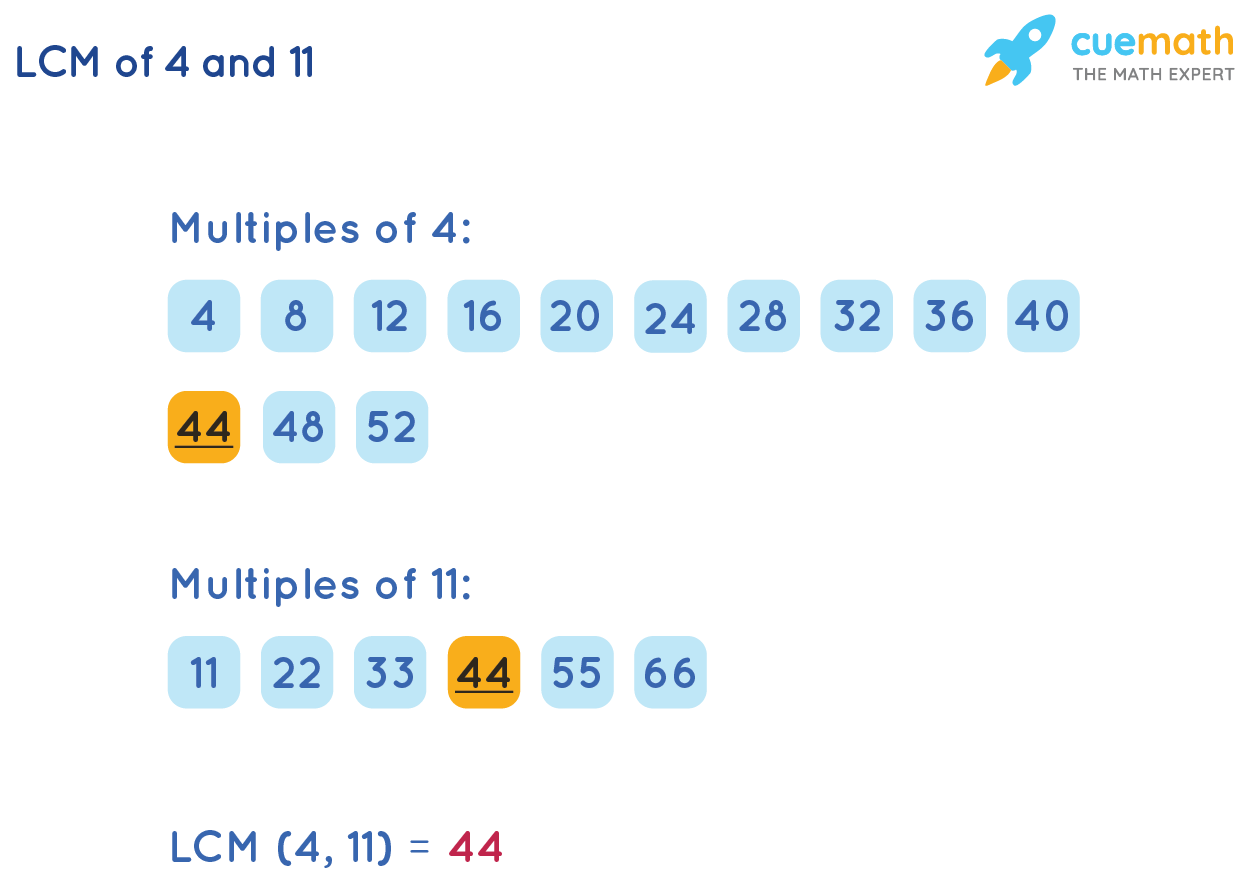# LCM of 4 and 11

## Question: What is The LCM of 4 and 11?

To find the LCM of 4 and 11, we need the least number which is exactly divisible by both 4 and 11.

## Explanation:

The Least Common Multiple (LCM) of two numbers a and b is the smallest number that divides a and b exactly.

We will explain two ways to find the LCM of 4 and 11.

### LCM of 4 and 11 by Formula

The formula of LCM is

LCM (a,b) = (a × b) / GCF (a,b)

The Greatest Common Factor (GCF) of (4, 11) is 1. So, GCF (4, 11) = 1

So, the LCM of 4 and 11 by using the formula,

LCM (4, 11) = (4 × 11) / 1

= 44/1

= 44

LCM of 4 and 11 = 44

### LCM of 4 and 11 by Listing Method

We will list the first few multiples of 4 and 11 and determine the common multiples.

The least among the common multiples is the LCM of 4 and 11Observe that the solution to our question LCM of 4 and 11 is the same in both methods.

### Thus, LCM of 4 and 11 is 44

SHEETS
GCF(HCF) - LCM Worksheet
GCF(HCF) - LCM Worksheet